This is a copy of a webpage.

The Series in Order
Universal Code: The Number of the numbers of the Mathematics
Code 19 and Creation
Cells and Code 19
Number 19 and Pascal Triangle
Prime Numbers and The Quran

The Sources.
Plichta Home
Universal Code: The Number of the numbers of the Mathematics
1Code 19 and Creation
2Code 19 and Creation
Cells and Code 19
Number 19 and Pascal Triangle
Prime Numbers and The Quran

## The special position of the number 19 in the construction of the fractal triangle.

Image 1: The Prime numbers in the fractal triangle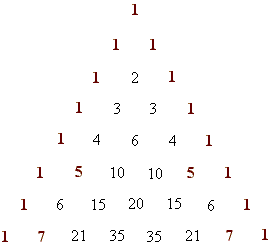The sum of the first nineteen digits of the fractal triangle  is 38 (or 2 * 19) :

Image 2: the first 19 digits.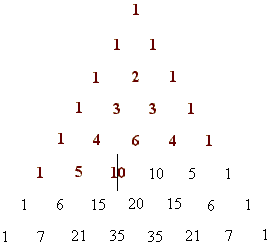The sum of the first nineteen numbers of the fractal triangle is 57 or (3 *19) :

Image 3: The first 19 numbers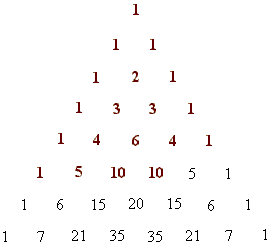The sum of the Prime numbers of a side of the fractal triangle is again 19:

Image 4: The sum of the Prime numbers of a side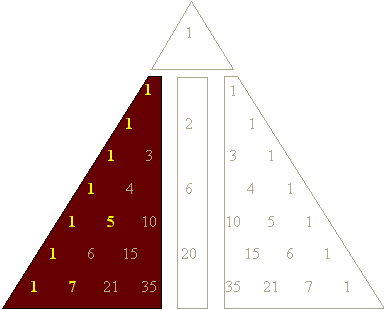By the first places of the numbers, there are exactly 19 'ones' :

Image 5: The digit 1 in the first places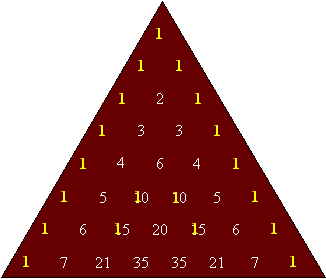Conclusion

Nineteen Prime numbers

The sum of the first nineteen digits is a multiple of 19

The sum of the first nineteen numbers is a multiple of 19

The sum of the prime numbers of a side is 19, and

Nineteen 'ones' are in the first places.

The key for the order of the Suras

The numbers of the fractal triangle reflects the position of the Suras

1. First revelation : sura 96 which is the 19th sura beginning to count from the end of the Quran consists of 19 verses of 285 letters (19*15) , and the first five verses of the revelation consist of 76 letters (19*4).

2. Second revelation : sura 68 . The first verses which were revealed consist of 38 or (19 * 2) words.

3. Third revelation : sura 73 which consist of 57 or (3 * 19) words.

4. The fourth revalation presents explicitly the number 19 and explain clearly that 19 is a Quranic code.

·       First  let's, examine in the fractal triangle, the numbers of their divisibility.

·      second, we determine the digits of the numbers.

·      third, we look which of  the numbers are even or odd.

We take the fractal triangle as basis and calculate the sum of all numbers which are divisible by 3. The result surprises: the sum is 96:

Illustration 1 : sum that through 3 divisible numbers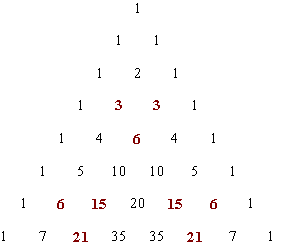The sum of the  numbers which are divisible by 3 is 96 - identical with the position of the first revealed Sura

The first revelation, that Mohammed p.u.h got, had not been classified by chance as the 96th Sure. It had a good reason: because the divisibility of the fractal triangle by 3 gives the position of the first revelation.

Divisions by 3 and by through 2 are the most familiar division-attempts. Since the Prophet, like God, loved the odd more than the even numbers, we started with numbers which are  divisible by 3.

Now let's look for the sum of all numbers which are divisible by 2 :

Illustration 2: sum of the  numbers which are divisible by 2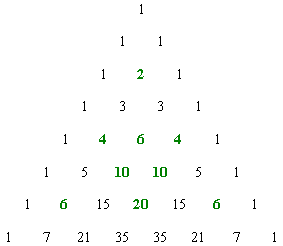The sum numbers which are divisible by 2 is 68 - identical with the position of the second revealed Sura

Also here the result surprises. It is 68. Is it a renewed coincidence ? We must negate the question. The selection of the positions of the first revelations seems to be mathematically justified and, to be incorporated in the fractal triangle.

The same is applied also to the third revelation, sure 73. How ? Let's add up the one-digit numbers of this triangle, and therefore the third positioning sura is clarified:

Illustration 3: sum of the one-digit numbers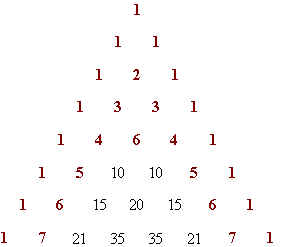The sum of the one-digit numbers is 73 - identical with the position of the 3rd  revealed Sura.

To be sure that there is no coincidence between the fractal triangle and the order of the revealed suras let's  find the number 74. which is the 4th revealed sura and where the number19 occurs for the first time  :

Illustration 4: sum of the mixed-digits numbers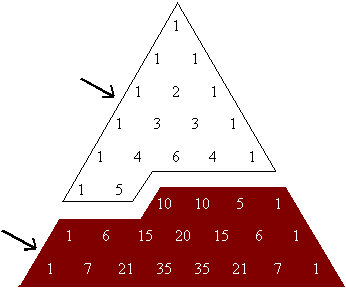1-  17 purely one-digit numbers gives a digit-sum of 37.

2-  19 mixed digits numbers give a digit-sum of 74 or 2 times 37

The sum of the digits of the remaining nineteen mixed digits numbers is 74 - identical with the position of the fourth revealed Sure.

Also the order of the first revelations derives from the fractal triangle.  The position of the first revelation (96) in the Quran in which is the sum of the numbers divisible by 3, the position of the second revelation (68) which is the sum of the numbers divisible by 2, and the position of the third revelation (73) which the sum of the one-digit numbers, are namely derived from  the fractal triangle.Finally the position of the fourth revelation is now determined by the sum of the digits of the nineteen 'mixed-digits' numbers, which come after only seventeen 'one-digit' numbers. We see here that number 74 is directly linked with these 19 digits exactly like sura 74 where the number 19 occurs for the first time. The parallels are astonishing.

The consequence of these simple reckonings are extremely stringent. The analysts too, who may extremely critically approach  the numerical structure of the Quran, will have to admit the significance of these calculations. Whereas believers will come to the certitude through the conspicuous agreement of these reckonings-operations of the Quranic numbers, that God has revealed to his beloved Messenger a mathematically structured  Quran.

Note : Pascal was born on June 19, 1623 and died on August 19, 1662.

To be continued inshallah......

more about triangle of Pascal :

from Stefan Makowski : " Worldformula 19"